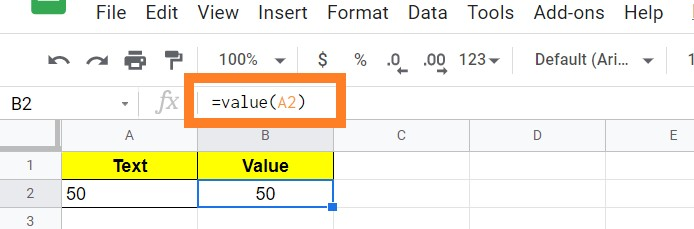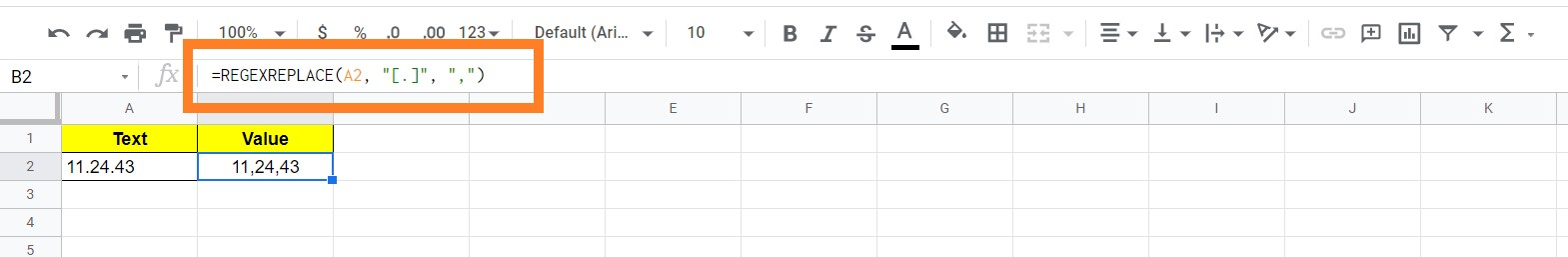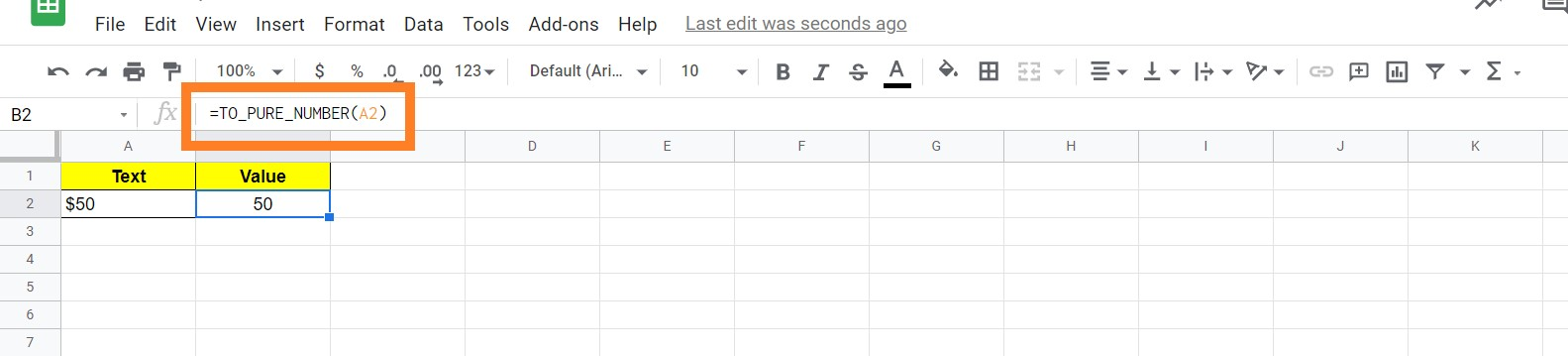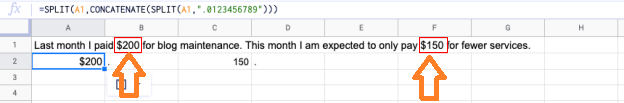# How To Convert Text to Numbers in Google Sheets: String to Text Conversion

Convert String to Number Formula: When you are working in Google Sheets, you can easily perform the mathematical operations when you are dealing with numbers. However, the same mathematical operations couldn’t be performed when it comes to the text nature of numbers. Thus to overcome this issue, we should convert the text to numbers in Google Sheets.

On this page, we have provided all the necessary information on how to convert the text to numbers. Read on to find more.

Also, check our article on Google Sheet Tips which are extremely useful and save a lot of time.

## Google Sheets Value Function to Convert Text to Numbers

With the help of the value function, we can easily convert the text to numbers. Let’s understand the conversion of text to numbers with the help of the following example.

• Step 1: Select the cell in Google Sheet, where you would like to make the conversion.
• Step 2: Enter the formula =value(text cell number).
• Step 3: You will see the results.Here our formula is =value(a2)

### Replace Dots with Comma to Convert Text to Numbers

Let’s consider you have received a dataset where the numbers are formatted with the help of “.” and other periods instead of commas. When the “.” and other periods are given in between the numbers, the G Sheet considers it as text instead of numbers.

For example, you have received a dataset where the number is represented as 112.443. The number “112.443” is considered as text in Google Sheet since it is formatted with the help of “.”. Thus to overcome this issue and convert these kinds of numbers into texts, follow the steps listed below:

• Step 1: Select the cell.
• Step 2: Enter the formula “=REGEXREPLACE(A2, “[.]”, “,”)“.
• Step 3: Press “Enter“. You will see the results### Converting Data with Currency to Number

You can easily convert the data which is substituted with a currency symbol into text by following the steps given below:

• Step 1: Select the text.
• Step 2: Enter the formula “=TO_PURE_NUMBER(A2)“.
• Step 3: Press “Enter“.

You will see the results as shown below.### Extracting Numbers from Text

In some instances, we would have received where both the numbers and text are combined in sentences. Google Sheets provides us with a great opportunity where we can easily extract the text into numbers.

The steps to extract the numbers from the text are explained below:

• Step 1: Select the cell where the text needs to be extracted.
• Step 2: Now enter the formula “=SPLIT(A1,CONCATENATE(SPLIT(A1,”.0123456789″)))
• Step 3: Press “Enter“.

You will see the results.In order to extract numbers from text, you can also make use of the following formula as shown below:

=SPLIT(REGEXREPLACE(A1, “[^\d\.]+”, “|”), “|”)